# How to Solve One-Step Equations

1. Math Lessons >
2. One-step Equations

What is a one-step equation?

The term “one-step equation” is self-explanatory. An equation that can be solved in a single-step is called a one-step equation. Have a look at the below equation:

c + 7 = 14

With just one unknown variable: c, this is an example of a one-step equation; all you need to do in order to solve this equation is to find the value of the variable. In other words, such equations are solved in one step.

In this lesson, we are going to walk you through the below-listed topics:

How do you solve a one-step equation?

To solve a one-step equation, you have to isolate the variable. To isolate the variable, use inverse operations.

Operations that undo each other are called inverse operations. They are:

b. Multiplication and Division

Let's now delve into the concept with the following examples.

How to Solve One-step Equations using Addition

To solve the equation involving subtraction, use the addition property of equality. Add the same number on both sides of the equation to balance the equality of the statement.

(i) Solve x − 9 = 15

The inverse of subtraction is addition.

x − 9 = 15

Add 9 on both sides of the equation.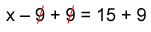Simplify the equation.

x = 24

How to Solve One-step Equations using Subtraction

As subtraction is the inverse of addition, the addition property of equality also holds for subtraction. To solve the equation involving addition, subtract the same numeral on both sides of the equation to balance the equality of the statement.

(ii) Solve x + 9 = 15

x + 9 = 15

Subtract 9 on both sides of the equation.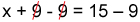x = 6

Plug-in the value of the variable in the equation to check your answer.

For the equation x + 9 = 15, substitute x = 6,

x + 9 = 15

6 + 9 = 15

15 = 15

How to Solve One-step Equations using Multiplication

To solve the equation involving division, multiply the same number to both sides of the equation to balance the equality of the statement; in other words, apply the multiplication property of equality.

(iii) Solve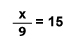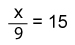Multiply 9 on each side of the expression.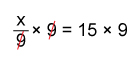x = 135

How to Solve One-step Equations using Division

As division is the inverse of multiplication, the multiplication property of equality also holds for division.

To solve an equation involving multiplication, divide the same number to both sides of the equation to maintain the equality of the statement.

(iv) Solve 9x = 15

9x = 15

Perform the inverse of multiplication (division) to remove the coefficient of x.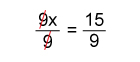x = 53

Real-life example:

1. Oliver has a great collection of toys. He gave 8 toys from his collection to Oscar as a gift. If he is left with 32 toys, how many toys did Oliver initially have?

The above scenario gives rise to an equation: x – 8 = 32, where x represents the number of toys Oliver initially had.

Add 8 on both sides of the equation,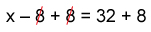x = 40

So, Oliver initially had 40 toys.

The lesson in a nutshell:

To obtain the value of a variable, you need to isolate the variable.

Perform inverse operations to isolate the variable. Remember to perform inverse operations on both sides of the equation.

Simplify the equation and find the value of the variable.

Check your answer by substituting the value of the variable in the equation.Solved Examples:

Example 1

1. Solve r + 10 = 21

r + 10 = 21

r + 10 − 10 = 21 − 10

[ Applying inverse operation on both sides ]

r = 11

Example 2

2. Solve h − 2 = −14

h − 2 = −14

h − 2 + 2 = −14 + 2

[ Adding 2 on both sides ]

h = −12

Example 3

3. Solve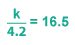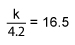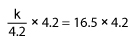[ Multiplying 4.2 on both sides ]

k = 69.3